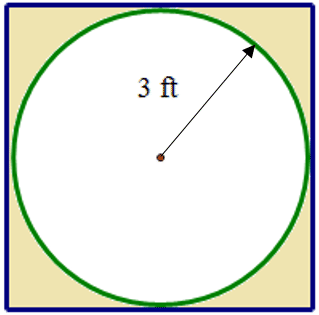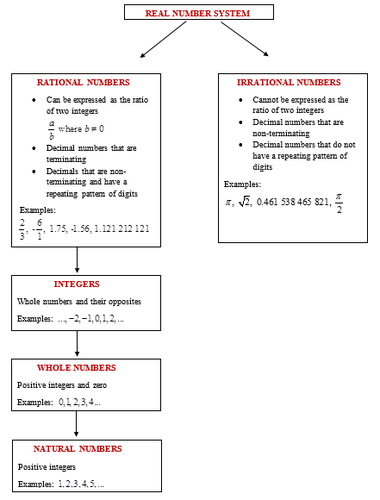# 1.1: Real Numbers

•• Contributed by CK12
• CK12

## Classify Real Numbers[Figure 1]

Faith has been planting a square flower bed for many years and has decided that the bedding plants are getting too expensive to fill such an area. This year she is going to plant a circular flower bed within the original square area as shown below:[Figure2]

As Faith reviews her plan, she has decided to find some exact measurements so she can figure out just how much smaller the new circular flower bed will be compared to the original square flower bed. However, Faith is unsure of what measurements she has to figure out – “I hope I at least come out with some rational numbers!”

In this concept, you will learn to classify real numbers.

### Real Numbers

All numbers belong to the set of numbers known as the real number system. The real number system consists of every number you have ever dealt with since you were old enough to count. The numbers in the real number system are divided into two main groups. One group is called the rational numbers and the other is called the irrational numbers. The set of irrational numbers consists of all numbers that are not rational. This set of irrational numbers includes those numbers that cannot be written as the ratio of two integers, decimal numbers that are non-terminating and decimals that do not have a repeating pattern of digits. For example, pi(π),√2,−2.345876921… are irrational numbers.

The set of rational numbers includes natural numbers, whole numbers, integers, numbers that can be expressed as the ratio of two integers, decimal number that terminate and decimal numbers that have a repeating pattern of digits. The natural numbers are the set of positive integers. For example, 1, 2, 3… are all natural numbers. The whole numbers are the natural numbers and zero. For example, 0, 1, 2, 3… are all whole numbers. The integers are the whole numbers and their opposites. For example, -2, -1, 0, 1, 2… are all integers. A rational number is any number that can be expressed in the form ab where b≠0. When a rational number is expressed as a decimal then the decimal will terminate (end) or it will have a repeating pattern of digits. For example, 1/2,√81,−7.456545654 are all rational numbers.

When you classify numbers remember that they can often belong to more than one set of numbers. If you think of the number 3, you can call it a natural number, a whole number, an integer and a rational number.

The following table may help you to better understand the real number system.[Figure3]

### Examples

#### Example 1

Earlier, you were given a problem about Faith and her circular flower bed. She wants to compare the new circular flower bed to the original square one using exact measurements. First, Faith should compare the perimeter of the square to the circumference of the circle. Then, she should compare the area of the square to the area of the circle.

First, determine the diameter of the circle which will be the side length of the square.

The diameter of a circle is two times the length of the radius. The radius of the circle is 3 feet.

d=2r

d=2(3)

d=6

The diameter of the circle is 6 feet. Each side of the square is also 6 feet in length.

The answer of 6 is a natural number, a whole number, an integer and a rational number.

Next, determine the perimeter of the square. The perimeter of the square is the distance around its outer edges and can be found by adding the lengths of each side or by simply multiplying the side length by four.

Psquare=4(s)

Psquare=4(6)

Psquare=24

The perimeter of the square is 24 feet.

The answer of 24 is a natural number, a whole number, an integer and a rational number.

Next, determine the circumference of the circle. The perimeter of a circle is known as its circumference and is the distance around the outer edge of the circle. The perimeter of a circle can be found by multiplying the diameter by π.

C=πd

C=(3.141592654)(6)

C=18.84955592

Using the value for π from the calculator (3.141592654) gives the answer 18.84955592.

The circumference of the circle is 18.84955592 feet.

The answer of 18.84955592 is an irrational number. It is a non-terminating, non-repeating decimal.

Next, determine the area of the square. The area of the square can be found using the formula:

A=s2 where ‘s’ is the side length of the square.

A=s2

A=(6)2

A=36

The area of the square flower bed is 36 ft2.

The answer of 36 is a natural number, a whole number, an integer and a rational number.

Next, determine the area of the circle. The area of the circle can be found using the formula:

A=πr2 where ‘r’ is the radius of the circle.

A=πr2

A=(3.141592654)(3)2

A=(3.141592654)(9)

A=28.27433388

The area of the circular flower bed is 28.27433388 ft2.

Using the value for π from the calculator (3.141592654) gives the answer 28.27433388.

The answer of 28.27433388 is an irrational number. It is a non-terminating, non-repeating decimal.

Next, subtract the area of the circular flower bed from the area of the square flower bed. Round the area of the circular flower bed to the nearest tenth and then perform the subtraction.

The difference in the area of the two flower beds is 36.0 ft2−28.3 ft2=7.7 ft2

The answer of 7.7 is a rational number since it is a terminating decimal.

#### Example 2

Let’s look at classifying some numbers.

For each of the following numbers displayed in the table, indicate the number set or sets to which they belong.

 Number Natural Whole Integer Rational Irrational 22/7 ⋆ 1.141 141 114… ⋆ 17/4 ⋆ -6 ⋆ ⋆ π/2 ⋆ 0 ⋆ ⋆ ⋆ 9 ⋆ ⋆ ⋆ ⋆

22/7 is a rational number because it is written as the ratio of two integers.

1.141 141 114… is an irrational number because it is a non-terminating decimal.

17/4 is a rational number because it is written as the ratio of two integers.

-6 is an integer because it is the opposite of the whole number 6. It is a rational number because it can be expressed as the ratio of two integers as −61.

π/2 is an irrational number because pi is a non-terminating decimal and is an irrational number.

0 is a whole number, an integer and a rational number. Zero is included in each of these number sets.

9 is a natural number, a whole number, an integer and a rational number. It is included in each of these sets and can be expressed as the ratio of two integers as 91.

The following table may help you to understand the real number system.

#### Example 3

For each of the following statements state if they are sometimes true, always true, or never true.

1. Whole numbers are integers.
2. Integers are whole numbers.
3. If a number is an integer, then it is also a rational number.
4. The number 3π is a rational number.
5. A number can be both rational and irrational
6. If a number is rational, then it is also an integer.

First, read each statement and review what numbers are included in each of the number sets.

a) The numbers that belong to the set of integers are the whole numbers and their opposites.

Therefore it is always true that the whole numbers are integers.

b) Integers are only whole numbers if the numbers are positive whole numbers or zero. Integers that are negative do not belong to the set of whole numbers.

Therefore it is sometimes true that integers are whole numbers.

c) The set of rational numbers includes any number that can be expressed as the ratio of two integers.

Therefore it is always true that if a number is an integer, then it is also a rational number.

d) The value of π expressed as a decimal is a non-terminating decimal with no repeated pattern of digits. π belongs to the set of irrational numbers.

Therefore it is never true that 3π is a rational number.

e) A number that belongs to the set of rational numbers cannot belong to the set of irrational numbers. Any number that cannot be written as a rational number is an irrational number.

Therefore it is never true that a number can be both rational and irrational.

f) If a number is rational, then it is also a natural number or a whole number or an integer.

Therefore, it is sometimes true that if a number is rational, then it is an integer.

#### Example 4

For each of the following numbers displayed in the table, indicate the number set or sets to which they belong. Justify your answer.

First, review what numbers are included in each of the number sets.

Remember a number can belong to one or more than one number set.

 Number Natural Whole Integer Rational Irrational Justification 6.18 Terminating decimal 0.89563... ⋆ Non-terminating decimal 12 ⋆ ⋆ ⋆ ⋆ Can be expressed as +12,12,12/1 -7 ⋆ ⋆ Can be expressed as −7,−7/1 −5/9 ⋆ fraction √36 ⋆ ⋆ ⋆ ⋆ Value is 6 −√5.5 ⋆ Non-repeating, non-terminating decimal 1/3 ⋆ fraction

### Review

Classify each of the following numbers as real, whole, integer, rational or irrational. Some numbers will have more than one classification.

1. 3.45

2. -9

3. 1270

4. 1.232323

5. 4/5

6. -232 323

7. -98

8. 1.98

9. √16

10. √2

State whether the following statements are true or false.

11. An irrational number can also be a real number.

12. An irrational number is a real number and an integer.

13. A whole number is also an integer.

14. A decimal is considered a real number and a rational number.

15. A negative decimal can still be considered an integer.

16. An irrational number is a terminating decimal.

17. A radical is always an irrational number.

18. Negative whole numbers are integers and are also rational numbers.

19. Pi is an example of an irrational number.

20. A repeating decimal is also a rational number.